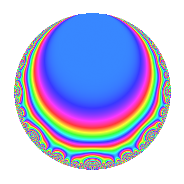# Properties

 Label 164.2.aLevel 164 Weight 2 Character orbit a Rep. character $$\chi_{164}(1,\cdot)$$ Character field $$\Q$$ Dimension 4 Newform subspaces 1 Sturm bound 42 Trace bound 0

# Related objects

## Defining parameters

 Level: $$N$$ = $$164 = 2^{2} \cdot 41$$ Weight: $$k$$ = $$2$$ Character orbit: $$[\chi]$$ = 164.a (trivial) Character field: $$\Q$$ Newform subspaces: $$1$$ Sturm bound: $$42$$ Trace bound: $$0$$

## Dimensions

The following table gives the dimensions of various subspaces of $$M_{2}(\Gamma_0(164))$$.

Total New Old
Modular forms 24 4 20
Cusp forms 19 4 15
Eisenstein series 5 0 5

The following table gives the dimensions of the cuspidal new subspaces with specified eigenvalues for the Atkin-Lehner operators and the Fricke involution.

$$2$$$$41$$FrickeDim.
$$-$$$$+$$$$-$$$$4$$
Plus space$$+$$$$0$$
Minus space$$-$$$$4$$

## Trace form

 $$4q + 2q^{3} + 4q^{5} + 12q^{9} + O(q^{10})$$ $$4q + 2q^{3} + 4q^{5} + 12q^{9} + 4q^{11} - 10q^{15} - 4q^{17} + 6q^{19} - 12q^{23} + 12q^{25} - 10q^{27} - 4q^{29} - 8q^{31} - 20q^{33} - 26q^{35} + 16q^{37} - 24q^{39} - 4q^{41} + 4q^{43} + 4q^{45} - 6q^{47} + 16q^{49} - 4q^{51} - 16q^{53} - 2q^{55} + 4q^{57} + 12q^{59} + 24q^{61} - 10q^{63} + 4q^{65} + 28q^{67} - 28q^{69} - 2q^{71} + 8q^{73} + 30q^{75} + 8q^{77} - 18q^{79} + 28q^{81} - 12q^{83} + 32q^{85} + 44q^{87} + 4q^{89} + 36q^{91} - 28q^{93} + 14q^{95} + 16q^{97} + 58q^{99} + O(q^{100})$$

## Decomposition of $$S_{2}^{\mathrm{new}}(\Gamma_0(164))$$ into newform subspaces

Label Dim. $$A$$ Field CM Traces A-L signs $q$-expansion
$$a_2$$ $$a_3$$ $$a_5$$ $$a_7$$ 2 41
164.2.a.a $$4$$ $$1.310$$ 4.4.25808.1 None $$0$$ $$2$$ $$4$$ $$0$$ $$-$$ $$+$$ $$q+(-\beta _{1}+\beta _{2})q^{3}+(2-\beta _{2}+\beta _{3})q^{5}+\cdots$$

## Decomposition of $$S_{2}^{\mathrm{old}}(\Gamma_0(164))$$ into lower level spaces

$$S_{2}^{\mathrm{old}}(\Gamma_0(164)) \cong$$ $$S_{2}^{\mathrm{new}}(\Gamma_0(41))$$$$^{\oplus 3}$$$$\oplus$$$$S_{2}^{\mathrm{new}}(\Gamma_0(82))$$$$^{\oplus 2}$$